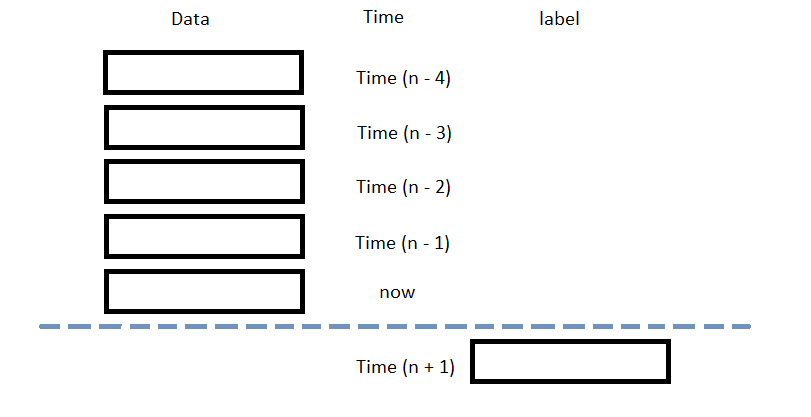# Popular Libraries

## XGBoost

### Introduction

This page explains how to build, train, deploy and store XGBoost models.

### Import Libraries

Import the XGBoost and joblib libraries.

from AlgorithmImports import *
import xgboost as xgb
import joblib

You need the joblib library to store models.

### Create Subscriptions

In the Initialize method, subscribe to some data so you can train the xgboost model and make predictions.

self.symbol = self.AddEquity("SPY", Resolution.Daily).Symbol

### Build Models

In this example, build a gradient boost tree regression prediction model that uses the following features and labels:

Data CategoryDescription
FeaturesThe last 5 closing prices
LabelsThe following day's closing price

The following image shows the time difference between the features and labels:### Train Models

You can train the model at the beginning of your algorithm and you can periodically re-train it as the algorithm executes.

#### Warm Up Training Data

You need historical data to initially train the model at the start of your algorithm. To get the initial training data, in the Initialize method, make a history request.

training_length = 252*2
self.training_data = RollingWindow[float](training_length)
self.training_data.Add(trade_bar.Close)

#### Define a Training Method

To train the model, define a method that fits the model with the training data.

def get_features_and_labels(self, n_steps=5):
close_prices = np.array(list(self.training_data)[::-1])
df = (np.roll(close_prices, -1) - close_prices) * 0.5 + close_prices * 0.5
df = df[:-1]

features = []
labels = []
for i in range(len(df)-n_steps):
features.append(df[i:i+n_steps])
labels.append(df[i+n_steps])

features = np.array(features)
labels = np.array(labels)
features = (features - features.mean()) / features.std()
labels = (labels - labels.mean()) / labels.std()

d_matrix = xgb.DMatrix(features, label=labels)

return d_matrix

def my_training_method(self):
d_matrix = self.get_features_and_labels()
params = {
'booster': 'gbtree',
'colsample_bynode': 0.8,
'learning_rate': 0.1,
'lambda': 0.1,
'max_depth': 5,
'num_parallel_tree': 100,
'objective': 'reg:squarederror',
'subsample': 0.8,
}
self.model = xgb.train(params, d_matrix, num_boost_round=10)

#### Set Training Schedule

To train the model at the beginning of your algorithm, in the Initialize method, call the Train method.

self.Train(self.my_training_method)

To periodically re-train the model as your algorithm executes, in the Initialize method, call the Train method as a Scheduled Event.

# Train the model every Sunday at 8:00 AM
self.Train(self.DateRules.Every(DayOfWeek.Sunday), self.TimeRules.At(8, 0), self.my_training_method)

#### Update Training Data

To update the training data as the algorithm executes, in the OnData method, add the current TradeBar to the RollingWindow that holds the training data.

def OnData(self, slice: Slice) -> None:
if self.symbol in slice.Bars:
self.training_data.Add(slice.Bars[self.symbol].Close)

### Predict Labels

To predict the labels of new data, in the OnData method, get the most recent set of features and then call the predict method.

new_d_matrix = self.get_features_and_labels(df)
prediction = self.model.predict(new_d_matrix)
prediction = prediction.flatten()

You can use the label prediction to place orders.

if float(prediction[-1]) > float(prediction[-2]):
self.SetHoldings(self.symbol, 1)
else:
self.SetHoldings(self.symbol, -1)

### Save Models

Follow these steps to save xgboost models into the Object Store:

1. Set the key name of the model to be stored in the Object Store.
2. model_key = "model"
3. Call the GetFilePath method with the key.
4. file_name = self.ObjectStore.GetFilePath(model_key)

This method returns the file path where the model will be stored.

5. Call the dump method the file path.
6. joblib.dump(self.model, file_name)

If you dump the model using the joblib module before you save the model, you don't need to retrain the model.

You can load and trade with pre-trained xgboost models that saved in Object Store. To load a xgboost model from the Object Store, in the Initialize method, get the file path to the saved model and then call the load method.

def Initialize(self) -> None:
if self.ObjectStore.ContainsKey(model_key):
file_name = self.ObjectStore.GetFilePath(model_key)
self.model = joblib.load(file_name)

The ContainsKey method returns a boolean that represents if the model_key is in the Object Store. If the Object Store does not contain the model_key, save the model using the model_key before you proceed.

### Clone Example Algorithm

You can also see our Videos. You can also get in touch with us via Discord.